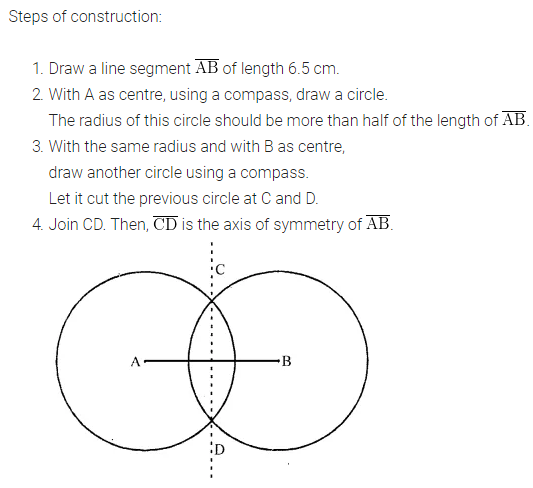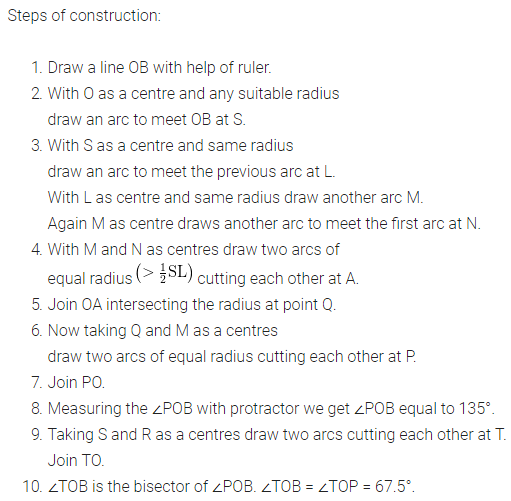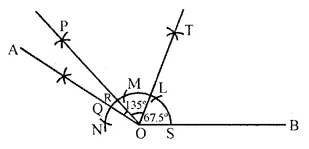ML Aggarwal Class 6 Solutions Chapter 13 Practical Geometry Check Your Progress for ICSE Understanding Mathematics acts as the best resource during your learning and helps you score well in your exams.

## ML Aggarwal Class 6 Solutions for ICSE Maths Chapter 13 Practical Geometry Check Your Progress

Question 1.
Draw a line segment AB = 5.4 cm. Construct a perpendicular at A by using ruler and compass.
Solution:
Steps of Construction:Question 2.
Draw a line segment PQ = 6.8 cm. Draw a perpendicular to it from a point A outside PQ by using ruler and compass.
Solution:Question 3.
Draw a line segment of length 6.5 cm and construct its axis of symmetry.
Solution:Question 4.
Draw ∠AOB = 76° with the help of a protractor. Bisect this angle by using a ruler and compass. Measure the two parts by your protractor and see how accurate you are.
Solution:
Steps of Construction:Question 5.
By using and compass, construct an angle of 135° and bisect it. Measure any one part by protractor and see how accurate you are.
Solution: